Comparative Study between the Darcy-Brinkman Model and the Modified Navier-Stokes Equations in the Case of Natural Convection in a Porous Cavity

Razli MEHDAOUI*, Mohammed ELMIR*, Belkacem DRAOUI*, Omar IMINE**, Abdelkader MOJTABI***

*Vibration & Thermal Laboratory, University of Bechar, PO Box 417, Bechar, Algeria.

**Faculty of Mechanical Engineering, U.S.T.Oran, Algeria.

***IMFT, UPS, 118 route de Narbonne, 31062, Toulouse cedex, France.

Abstract

The objective of this study is the comparison between the model of Darcy-Brinkman and the Navier-Stokes equations modified, in the case of the natural convection. The study is made in a porous vertical square cavity saturated by a Newtonian fluid. A cylindrical heat source maintained at a uniform heat flux is introduced into porous medium. The equations which describe the thermal transfer and the hydrodynamic flow of the two models are solved numerically by means of the software package Femlab 3.2 based on the finite element method. The results obtained are in the form of average kinetic energy per unit mass, the local and the average Nusselt numbers, the pressure and the viscous force per unit area.

Keywords

Natural convection; Porous; Saturated; Darcy-Brinkman; Femlab 3.2.

Introduction

Natural convection in porous media is of practical interest in several sciences, engineering, agriculture; energy’s stocking system, building, geothermal science, medical and biological sciences. We note that in the theoretical domain, several works related to natural convection in porous media was been examined. We quote those of Nield , Cheng , Combarnous and Bories . We also find the problems of thermal sources flooded in a porous media studied by Bejan , Hickos  and Polikakos & Bejan . An important number of studies of natural convection in confined and semi-confined porous media, especially those of Bejan and Khair , Weber , Masuouka and al. . Convective processes of fluid flow and associated heat transfer in porous cavities have been studied extensively [10-12]. These studies focus on the thermal convection performance within a heated porous cavity for different geometrical parameters (aspect ratio), heating mode (isothermal) . The two dimensional free convection within a porous square cavity heated on one vertical side and cooled on the opposite side, while the horizontal walls are adiabatic, is currently considered a reference or benchmark solution for verifying other solution procedures. For a list of the basic references concerning this subject, we refer to the review articles by Ingham and Pop , Vafai , Pop and Ingham , Bejan and Kraus , Ingham and al. , Bejan and al. . The Brinkman-extended Darcy model has been considered by Tong and Subramanian , and Lauriat and Prasad  to examine the buoyancy effects on free convection in a vertical cavity. Eungsoo and al.  have examined the non-Darcy effects of the natural convection in porous cavity.

In this fundamental study, we compare the two models (Darcy-Brinkman and Navier-Stokes equations modified) and to visualize their influences on the natural convection in porous square cavity whose walls are isothermal. A cylindrical heat source maintained at a isoflux is introduced into porous media.

Physical and mathematical models

In the presence of a concentric cylindrical source of diameter D, submerged in porous media subjected to a uniform heat flow. We suggest studying the natural convection in a square cavity (Fig. 1) of side length H, in presence of concentric cylindrical source of diameter D, submerged in porous media subjected to a uniform heat flux q. The walls of cavity are kept at uniform temperature Tw.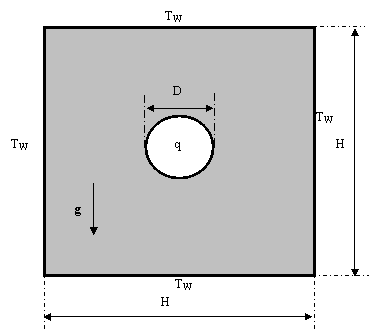Figure 1. Physical model

We suppose that the porous media is saturated, the flow is laminar, two-dimensional and the fluid is Newtonian. The properties of the fluid and the porous media are constants. The viscous dissipation and the radiation are neglected. The Boussinesq’s approximation is valid.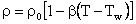The flow field is governed by the Darcy-Brinkman equation, the Navier-Stokes equation modified by using the extension of Darcy-Brinkman , and the thermal field by the energy equation.

The volume-averaged conservation dimensionless equations of mass, Darcy-Brinkman, Navier-Stokes modified and energy are: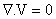(1)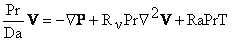(2)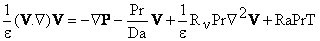(3)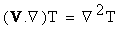(4)

The governing equations are made dimensionless by adopting the following dimensionless quantities: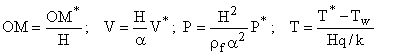In the above system, the following dimensionless numbers appear: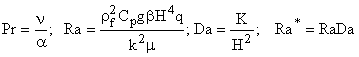k represents the thermal conductivity of porous media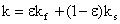, where f is fluid properties and s is solid properties

The hydrodynamic boundary conditions at express the impermeability and the no slip on the rigid walls and the contour of cylindrical source of the cavity. The external walls are maintained at uniform temperature all are adiabatic and the cylindrical source is maintained at uniform temperatures. These dimensionless boundary conditions are represented in table1:

Table 1. Boundary conditions

 Faces V T External walls 0 0 Concentric circular source 0 ∂T/∂n=1

The dimensionless average kinetic energy per unit mass is given by: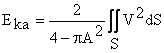(5)

where S is the dimensionless domain.

The average Nusselt number, on the circumference source is defined by(6)

where c is the dimensionless arc-length.

The dimensionless viscous stress, on the circumference source is determined by the relation: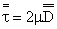, where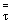is the tensor of the constraints and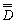the tensor of the rates of deformation.

Numerical method

Numerical results are obtained by solving the system of differential equations (1)–(4), with appropriate boundary conditions, using the Galerkin finite-element method implemented through the software package Femlab 3.2. The two-dimensional spatial domain is divided into triangular elements (unstructured mesh) and a Lagrange-quadratic interpolation has been chosen. The mesh is refined near the boundaries and we have adopted 818 elements and the number of freedom degree is equal to 5681 (Fig.2). A nonlinear solver has been used and the nonlinear tolerance has been set to 10-6.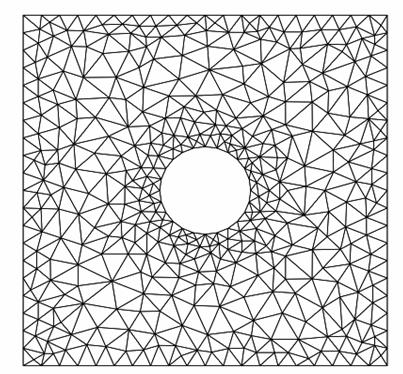Figure2. Mesh grid

Results and Discussion

To describe the flow structure of natural convection in the porous cavity, using the two models, the following parameters are fixed:

A=0.25, Da=0.002, Pr=0.71, e=0.513

The effective viscosity in the Brinkman’s term is equal to the fluid viscosity meff=m what corresponds to Rn =1.

 Ra*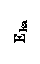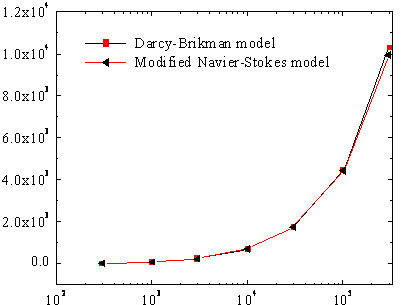Figure 3. Evolution of the average kinetic energy per unit of mass vs.

modified Rayleigh number

Figure 3 represents the evolution of the average kinetic energy per unit of mass in whole domain, for various values of modified Rayleigh number Ra*, by using the two models: Darcy-Brinkman and modified Naviers-Stokes.

The two curves evolve in the same way and believe with the Ra* increase. A light variation appears on the values between the two models for Ra* >105, which justifies the results of table1. For Ra* <104, the average kinetic energy Eka is almost null, thus giving a static pseudo flow that favours the conductive transfer mode. Beyond these values, the flow is carried out with velocities important enough, thus giving a dominating convective transfer mode.

The results obtained for the two models are in good agreement thus making it possible to neglect the influence of convective term (V.Ñ)V in the Naviers-Stokes equations (See Table 2).

Table 2. Average kinetic energy

 Ra* 300 3000 30000 3e5 Eka Da-Br 19.193 243.7 1724.6 10305 N.S.m 19.217 245.45 1737.4 9905 Error  (%) 0.12 0.72 0.74 3.95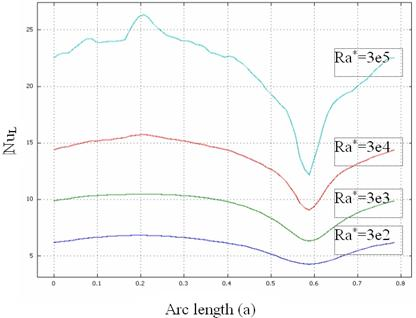Figure 4.a. Evolution of the local Nusselt number along the circumference of heat source - Da-Br model

Figure 4 (a and b) represents the evolution of the local Nusselt number along the circumference of heat source, for various values of Ra* and that for two models. The two curves evolve in the same way between two extremums (max, min). Nul increases with the Ra* increase. A bifurcation appears for Ra* > 3e3 with the position (Arc-length=0.6). With this position and for Ra*=3e5, the precision on local Nusselt number between the two models is about 10%. This precision decreases with the Ra* decrease.

 Arc length  (b)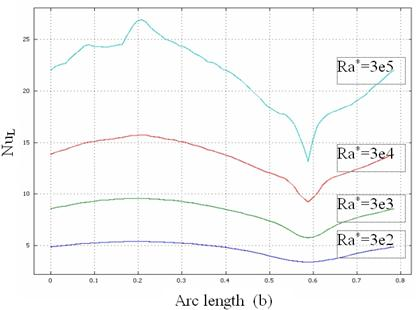Figure 4.b.  Evolution of the local Nusselt number along the circumference of heat source - N.S.m model

 Ra*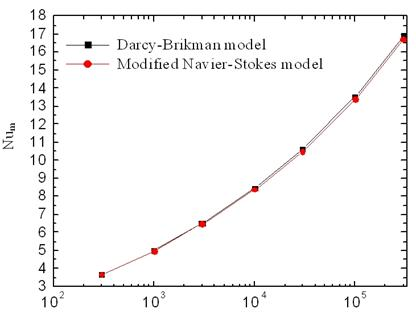Figure 5. Evolution of the average Nusselt number vs. modified Raleigh number

Figure 5 represents the variation of the average Nusselt number Num with various values of Ra* on the circumference of heat source. For the two models, Num evolves in the same way. For Ra*>1e4, a light variation on the values is distinguished. The values of Num increases with the Ra* increase allowing the increase in the rate of the convective transfer mode. The analysis of the values of Num deferred in table2 enables us to conclude that convective term (V.Ñ)V, in the Naviers-Stokes equation, does not have almost any influence.

Table 3. Average Nusselt number

 Ra* 300 3000 30000 3e5 Num Da-Br 3.646 6.498 10.61 19.92 N.S.m 3.647 6.474 10.47 16.72 Error  (%) 0.03 0.4 1.4 1.20

 Ra*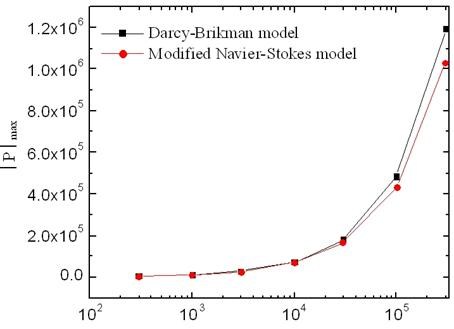Figure 6. Evolution of the maximum pressure in absolute value vs. modified Raleigh number

Figure 6 represents the variation of the maximum pressure in absolute value │P│max, according to Ra* on whole geometrical domain. Same remarks raised on the preceding figures. Except for Ra* >3e4, a difference in pressure about 2e5 is noted (see Table3).

Table 4. Maximum absolute pressure

 Ra* 3e2 3e3 3e4 3e5 │P│max Da-Br 0.55e5 2.83e5 1.80e5 11.9e5 N.S.m 0.55 e5 2.80 e5 1.69 e5 10.3 e5 Error  (%) 0.18 1.09 6.11 13.7

Figure7 (a and b) represents the evolution of the viscous stress along the circumference of heat source for various Ra* values. The pace of the evolution is the same one for the two models. For low Ra* values, the viscous stress are almost null, what confirms nature pseudo-statics of the flow thus giving a conductive mode transfer.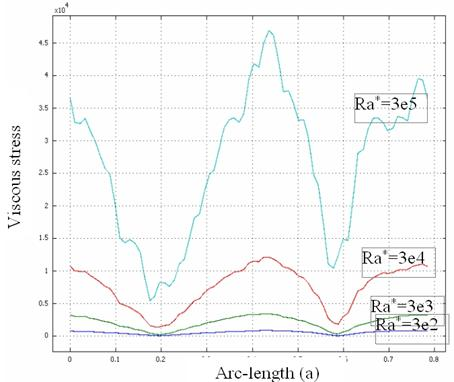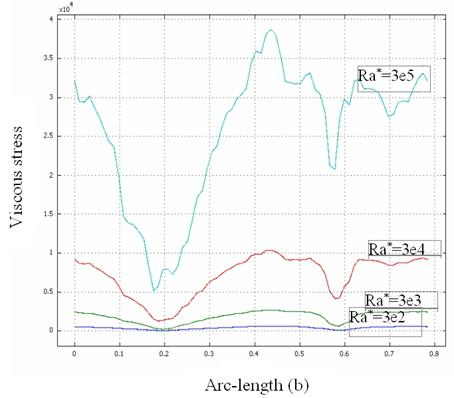Figure 7. Evolution of the viscous stress along the circumference of heat source

(a) Da-Br model - (b) N.S.m model

The curves present extremums (max, min) on the remarkable points of the circumference. The rate of deformation is important at the points (Arc-length=0.4; 0.8) where the shearing of the fluid is important. For high Ra* values the viscous stress are symmetrical in the Darcy-Brinkman model, on the other hand they lose their symmetry in the modified Navier-Stokes model. For the various Ra* values, the precision on the viscous stress is about 17%.

Conclusions

The natural convection in a porous vertical square cavity saturated by a Newtonian fluid is considered in this paper. A cylindrical heat source maintained at a uniform heat flux is introduced into porous medium. The walls of cavity are kept at uniform temperature Tw. The dimensionless forms of the continuity, Darcy-Brinkman equation, modified Navier-Stokes equations and the energy equation are solved numerically using the Galerkin finite-element method implemented through the software package Femlab 3.2.

From the results obtained in the form of average kinetic energy, average Nusselt number and pressure for various modified Rayleigh number values, the relative error between the two models does not exceed 6%. Except for Ra*=3e5 which corresponds with Ra=1.5e8 (limit of the laminar flow), the error on the pressure can reach 13%. One can conclude that the convective term (V.Ñ)V does not have almost any influence on the results of the two models.

For the grid considered and by using a CPU of processor 1.7 GHz and 2 Go of RAM. The solution time for Ra*=3e5 is of 82 seconds for modified Navier-Stokes model. On the other hand the solution time is of 65.5 seconds for the Darcy-Brinkman model. Therefore, the Darcy-Brinkman model allows a saving of time of 25% compared to that of modified Navier-Stokes model. It is preferable to use the Darcy-Brinkman model.

References

1.      Nield D A., The stability of convective flow in porous media, in convective heat and mass transfer in Porous Media, Serie E., Applied Science, Vol. 196, Kluuwer Academic, Dordrecht, 1991.

2.      Cheng P., Heat transfer in geothermal system, Adv. Heat Transfer, 1978, 14, p. 1-105.

3.      Combarnous and Bories, Hydrothermal convection in satured porous medium, Adv. Hydrosci, 1977, 10, p. 231-307.

4.      Bejan A., Natural convection in an infinite porous medium with a concentrated heat source, J. Fluid Mech, 1979, p. 89, p. 97-107.

5.      Hickox C.E., Thermal convection at low Rayleigh number from concentrated source in porous media, J. Heat Transfer, 1981, 103, p. 232-236.

6.      Polikakos D., Bejan A., Natural convection in vertically and horizontally layered porous media heated from the side international journal of heat and mass transfer, 1983, 26, p. 1805-1813.

7.      Bejan A., Khair K.R., Heat and mass transfer by natural convection in a porous medium, Int. J. heat Mass Transfer, 1985, 28, p. 909-918.

8.      Weber J.E., The boundary layer regime for convection in vertical porous layer, Int. J. Heat Mass Transfer, 1975, 18, p. 569-573.

9.      Masuouka T., Yokote Y., Katsuhara T., Heat transfer by natural convection in vertical porous layer, Bull. JSME, 1981, 24, p. 995-1001.

10.  Daniels P.G., Punpocha M., Cavity flow in a porous medium driven by differential heating, International Journal of Heat and Mass Transfer, 2004, 47, p. 3017-3030.

11.  Tanmay Basak, Roy S., Paul T., Pop I., Natural convection in a square cavity filled with a porous medium: Effects of various thermal boundary conditions, International Journal of Heat and Mass Transfer, 2006, 49, p. 1430-1441.

12.  Mehdaoui R., Elmir M., Imine O., Mojtabi A., Influence of the Darcy number on the natural convection in a partially porous enclosure, 3rd International Conference on Applications of Porous Media, May 29-June 3, 2006, Marrakech, Morocco.

13.  Mehdaoui R., Elmir M., Imine O., Mojtabi A., Influence of aspect ratio on natural convection in a partially porous enclosure, Journal of applied Sciences, Asian Network for scientific Information, 2006, 14, p. 2866-2874.

14.  Mehdaoui R., Elmir M., Imine O., Mojtabi A., Influence of the Darcy number on the natural convection in a partially porous enclosure, 3rd International Conference on Applications of Porous Media, May 29-June 3, 2006, Marrakech, Morocco

15.  Ingham D.B., Pop I., Transport Phenomena in Porous Media, Pergamon, Oxford, vol. III, 2005.

16.  Vafai K, Handbook of Porous Media, Marcel Dekker, New York, 2000.

17.  Pop I., Ingham D.B., Convective Heat Transfer, Mathematical and Computational Modelling of Viscous Fluids and Porous Media, Pergamon, Oxford, 2001.

18.  Bejan A., Kraus A.D., Heat Transfer Handbook, Wiley, New York, 2003.

19.  Ingham D.B., Bejan A., Mamut E., Pop I., Emerging Technologies and Techniques in Porous Media, Kluwer, Dordrecht, 2004.

20.  Bejan A., Dincer I., Lorente S., Miguel A.F. and Reis A H V, Porous and Complex Flow Structures in Modern Technologies, 2004, Springer, New York.

21.  Tong T.W., Subramanian E., Boundary layer analysis for natural convection in porous enclosure: use of the Brinkman-extended Darcy model, Int. J. Heat Mass Transfer, 1985, 28, p. 563-571.

22.  Lauriat G., Prasad V., Natural convection in a vertical porous cavity: a numerical study for Brinkman-extended Darcy formulation, Trans. ASME J. Heat Transfer, 1987, p. 109, 295-320.

23.  Eungsoo C., Chakma A., A bifurcation study of natural convection in porous media with internal heat sources: the non-Darcy effects, Int. J. heat mass transfer, 1997, 41(2), p. 383-392.

24.  Vafai K, Convective Flow and Heat Transfer in Variable-Porosity Media, J. Fluid Mech, 147, 1984, p. 233-259.

Nomenclature

 A D Da Da-Br Eka H K k N.S.m Nul Num OM* P* P* Aspect ratio; A = D/H [-]                                                                       Diameter of the cylinder source[m]                                       Darcy number [-] Darcy-Brinkman Average kinetic enrgy [-] Width of the square cavity [m] Permeability [m2] Thermal conductivity [w(mK)-1] Modified Navier-stokes Local Nusselt Number [-] Average Nusselt Number [-] Vector position  [m] Pressure [N.m-2] Pressure dimensionneless [-] Pr Ra Ra* Rn T* T V* V   a            e n μ (rfCp) Prandlt number [-] Rayleigh number [-] Modified Rayleigh number [-] Viscosity ratio; Rn = meff/mf [-] Temperature [K] Temperature dimensionless [-] Vector velocity [m.s-1] Vector velocity dimensionless [-] Greek symbols: Thermal diffusivity [m2.s-1] Porosity [-] Kinematic viscosity [m2.s-1] Dynamic viscosity [m-1.s-1] Heat capacity [j.m-3.K-1]